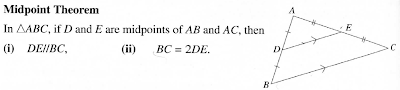### Geometrical Proofs

IntroductionIntercept theorem
There are 2 ways to apply the intercept theorem

In the first scenario, you will be able to identify a pair of parallel lines from the question
Upon identifying the pair of parallel lines,
we conclude that the ratio of AD:DB is the same as the ratio of AE:EC

In the second scenario, you identify that that the ratio of AD:DB is the same as the ratio of AE:EC
Subsequently you conclude that the lines DE and BC are parallelMidpoint Theorem
The Midpoint theorem is a specific case of the Intercept theorem when the ratio of the lengths is 1:1

There are 2 ways to apply the Midpoint Theorem

The first way is to use Midpoint Theorem to show that 2 lines are parallel
From the information given in the question, we will be able to identify that D is the midpoint of AB and E is the midpoint of AC
Given that D and E are midpoints, we will be able to conclude that DE is parallel to BC

The second way is to use Midpoint Theorem to show that E is a midpoint
From the information given in the question, we know that DE is parallel to BC and D is a midpoint
Using the Midpoint theorem, we can conclude that E is the midpoint of AC

QuestionGiven that:
O is centre of circle, AB is diameter, C lies on circle
AD is tangent to circle at A, EC is tangent to circle at C

i) Prove that triangles AEO and CEO are congruent
Strategy: make use of information given in the question
AD is tangent to circle --> angle OAE is 90 degrees
EC is tangent to circle --> angle OCE is 90 degrees
O is centre of circle --> OA and OC are radii of the circle, hence their lengths are equal
From diagram OE is the common length
Using the RHS property, we can show that triangles AEO and CEO are congruent

1.Hey, this is a great post, thank you so much for sharing. I’m looking forward to coming back to your site for more great information. Keep up the good work! : )

2.Hey, this is a great post, thank you so much for sharing. I’m looking forward to coming back to your site for more great information. Keep up the good work! : )

3.This comment has been removed by the author.

4.This information is meaningful and magnificent which you have shared here Math tuition. I am impressed by the details that you have shared in this post and It reveals how nicely you understand this subject. I would like to thanks for sharing this article here. Maths Tuition In Adelaide

5.It is truly a well-researched content and excellent wording. I got so engaged in this material that I couldn’t wait to read. I am impressed with your work and skill. Thanks.Online English Classes

6.7.Nice. I am really impressed with your writing talents and also with the layout on your weblog. Appreciate, Is this a paid subject matter or did you customize it yourself? Either way keep up the nice quality writing, it is rare to peer a nice weblog like this one nowadays. Thank you, check also event management and meeting thank you email

8.I just need to say this is a well-informed article which you have shared here about hoodies. It is an engaging and gainful article for us. Continue imparting this sort of info, Thanks to you. English Resources